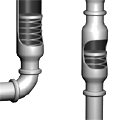jonathan footea book

I'm working on a book. Here's an introductory paragraph:

This is a book that explains electricity using intuition rather than equations. This book is different from other electronics books. It will give you a feel of how electricity works, using images and your physical intuition. With this, schematics and mathematics will make much more sense, and you will be able to solve textbook problems and design real circuits using your intuition. You will learn how electrical circuits behave using a fluid analogy that requires no mathematics. You will see some simple plumbing arrangements, and learn how electronic circuits can act just like them. Along the way, you will learn electronics terms and principles just by following along. Though this book is not a substitute for learning the theory, it will help you understand it, feel it, so you can better use it. The fluid analogy is surprisingly apt in nearly every case — even the equations would be exactly the same — so understanding it will give you a great head start. When you are done with this book, you will intuitively understand basic electronics. Not only that, but you will have picked up some deep concepts — conservation of energy, differential calculus — along the way.